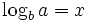Deutsche Version● Calculate logarithm

The logarithm of a value of an arbitrary base can
be calculated, as well as all inverse calculations.

Formula:, that means alsoThe logarithm of the number a in base b is the exponent x such that a = bx

 log 2 = lg, sometimes ld log e = ln or log log 10 = lg, sometimes log

Shortened spelling:

 log10 x = lg x loge x = ln x log2 x = lb x

Please enter two values, the third will be calculated.

 a (number): b (base): x (exponent): Decimal places:

The natural logarithm ln is, when the base b = e. Euler's number e = 2.718281828459...

What is the logarithm of 4? Answer 0.60206 (exponent). Base b = 10. log 4 = 0.60206
What number the has exponent x = 0.81292? Answer 6.5 (number). Base b = 10. 100.81292 = 6.5